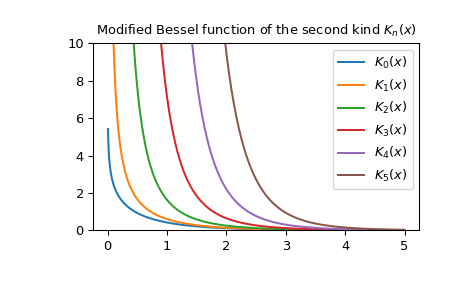# scipy.special.kn¶

scipy.special.kn(n, x) = <ufunc 'kn'>

Modified Bessel function of the second kind of integer order n

Returns the modified Bessel function of the second kind for integer order n at real z.

These are also sometimes called functions of the third kind, Basset functions, or Macdonald functions.

Parameters
narray_like of int

Order of Bessel functions (floats will truncate with a warning)

zarray_like of float

Argument at which to evaluate the Bessel functions

Returns
outndarray

The results

kv

Same function, but accepts real order and complex argument

kvp

Derivative of this function

Notes

Wrapper for AMOS  routine zbesk. For a discussion of the algorithm used, see  and the references therein.

References

1(1,2)

Donald E. Amos, “AMOS, A Portable Package for Bessel Functions of a Complex Argument and Nonnegative Order”, http://netlib.org/amos/

2(1,2)

Donald E. Amos, “Algorithm 644: A portable package for Bessel functions of a complex argument and nonnegative order”, ACM TOMS Vol. 12 Issue 3, Sept. 1986, p. 265

Examples

Plot the function of several orders for real input:

>>> from scipy.special import kn
>>> import matplotlib.pyplot as plt
>>> x = np.linspace(0, 5, 1000)
>>> for N in range(6):
...     plt.plot(x, kn(N, x), label='$K_{}(x)$'.format(N))
>>> plt.ylim(0, 10)
>>> plt.legend()
>>> plt.title(r'Modified Bessel function of the second kind $K_n(x)$')
>>> plt.show()Calculate for a single value at multiple orders:

>>> kn([4, 5, 6], 1)
array([   44.23241585,   360.9605896 ,  3653.83831186])


#### Previous topic

scipy.special.yve

scipy.special.kv